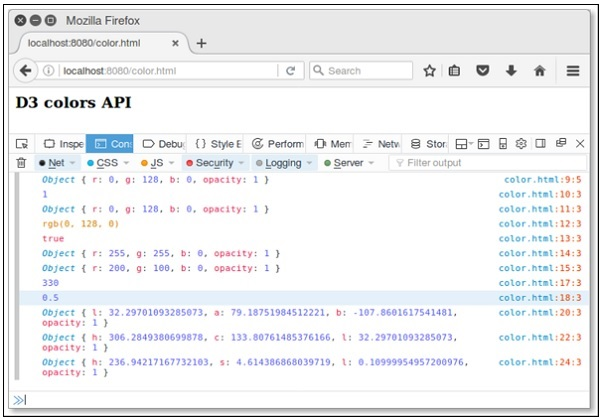# D3.js - Colors API

Colors are displayed combining RED, GREEN and BLUE. Colors can be specified in the following different ways −

• By color names
• As RGB values
• As HSL values
• As HWB values

The d3-color API provides representations for various colors. You can perform conversion and manipulation operations in API. Let us understand these operations in detail.

## Configuring API

You can directly load API using the following script.

```<script src = "https://d3js.org/d3-color.v1.min.js"></script>
<script>

</script>
```

## Basic Operations

Let us go through the basic color operations in D3.

Convert color value to HSL − To convert color value to HSL, use the following Example

```var convert = d3.hsl("green");
```

You can rotate the hue by 45° as shown below.

```convert.h + =  45;
```

Similarly, you can change the saturation level as well. To fade the color value, you can change the opacity value as shown below.

```convert.opacity = 0.5;
```

## Color API Methods

Following are some of the most important Color API Methods.

• d3.color(specifier)
• color.opacity
• color.rgb()
• color.toString()
• color.displayable()
• d3.rgb(color)
• d3.hsl(color)
• d3.lab(color)
• d3.hcl(color)
• d3.cubehelix(color)

Let us understand each of these Color API Methods in detail.

### d3.color(specifier)

It is used to parse the specified CSS color and return RGB or HSL color. If specifier is not given, then null is returned.

Example − Let us consider the following example.

```<script>
var color = d3.color("green");  // asign color name directly
console.log(color);
</script>
```

We will see the following response on our screen −

```{r: 0, g: 128, b: 0, opacity: 1}
```

### color.opacity

If we want to fade the color, we can change the opacity value. It is in the range of [0, 1].

Example − Let us consider the following example.

```<script>
var color = d3.color("green");
console.log(color.opacity);
</script>
```

We will see the following response on the screen −

```1
```

### color.rgb()

It returns the RGB value for the color. Let us consider the following example.

```<script>
var color = d3.color("green");
console.log(color.rgb());
</script>
```

We will see the following response on our screen.

```{r: 0, g: 128, b: 0, opacity: 1}
```

### color.toString()

It returns a string representing the color according to the CSS Object Model specification. Let us consider the following example.

```<script>
var color = d3.color("green");
console.log(color.toString());
</script>
```

We will see the following response on our screen.

```rgb(0, 128, 0)
```

### color.displayable()

Returns true, if the color is displayable. Returns false, if RGB color value is less than 0 or greater than 255, or if the opacity is not in the range [0, 1]. Let us consider the following example.

```<script>
var color = d3.color("green");
console.log(color.displayable());
</script>
```

We will see the following response on our screen.

```true
```

### d3.rgb(color)

This method is used to construct a new RGB color. Let us consider the following example.

```<script>
console.log(d3.rgb("yellow"));
console.log(d3.rgb(200,100,0));
</script>
```

We will see the following response on the screen.

```{r: 255, g: 255, b: 0, opacity: 1}
{r: 200, g: 100, b: 0, opacity: 1}
```

### d3.hsl(color)

It is used to construct a new HSL color. Values are exposed as h, s and l properties on the returned instance. Let us consider the following example.

```<script>
var hsl = d3.hsl("blue");
console.log(hsl.h + =  90);
console.log(hsl.opacity = 0.5);
</script>
```

We will see the following response on the screen.

```330
0.5
```

### d3.lab(color)

It constructs a new Lab color. The channel values are exposed as ‘l’, ‘a’ and ‘b’ properties on the returned instance.

```<script>
var lab = d3.lab("blue");
console.log(lab);
</script>
```

We will see the following response on the screen.

```{l: 32.29701093285073, a: 79.18751984512221, b: -107.8601617541481, opacity: 1}
```

### d3.hcl(color)

Constructs a new HCL color. The channel values are exposed as h, c and l properties on the returned instance. Let us consider the following example.

```<script>
var hcl = d3.hcl("blue");
console.log(hcl);
</script>
```

We will see the following response on the screen.

```{h: 306.2849380699878, c: 133.80761485376166, l: 32.29701093285073, opacity: 1}
```

### d3.cubehelix(color)

Constructs a new Cubehelix color. Values are exposed as h, s and l properties on the returned instance. Let us consider the following example.

```<script>
var hcl = d3.hcl("blue");
console.log(hcl);
</script>
```

We will see the following response on the screen,

```{h: 236.94217167732103, s: 4.614386868039719, l: 0.10999954957200976, opacity: 1}
```

### Working Example

Let us create a new webpage – color.html to perform all the color API methods. The complete code listing is defined below.

```<html>
<script type = "text/javascript" src = "https://d3js.org/d3.v4.min.js"></script>

<body>
<h3>D3 colors API</h3>
<script>
var color = d3.color("green");
console.log(color);
console.log(color.opacity);
console.log(color.rgb());
console.log(color.toString());
console.log(color.displayable());
console.log(d3.rgb("yellow"));
console.log(d3.rgb(200,100,0));
var hsl = d3.hsl("blue");
console.log(hsl.h + =  90);
console.log(hsl.opacity = 0.5);
var lab = d3.lab("blue");
console.log(lab);
var hcl = d3.hcl("blue");
console.log(hcl);
var cube = d3.cubehelix("blue");
console.log(cube);
</script>
</body>
</html>
```

Now, request the browser and we will see the following response.